Sie sind hier: ICP » R. Hilfer » Publikationen

# 5 Appendix: Proof of Proposition 2.2

[126.1.1] The proof given below follows Ref. . [126.1.2] Suppose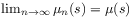and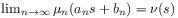with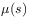and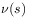both nondegenerate. [126.1.3] Then it must be shown that there exist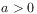and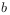such that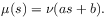(182)

[126.1.4] Pick a sequence of integers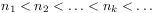such that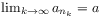and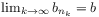exist with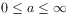and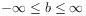. [126.1.5] Consider this sequence of indices from now on as fixed. [126.1.6] Then, to simplify the notation, suppose without loss of generality that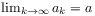and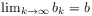.

[126.2.1] First it will be shown that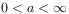. [126.2.2] Suppose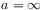. [126.2.3] Let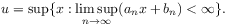(183)

[126.2.4] Then for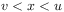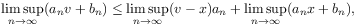(184)

and hence for every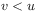it follows that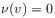because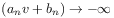with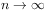. [126.2.5] For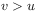, on the other hand,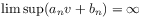and hence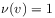for. [126.2.6] Thus the assumptioncontradicts tobeing nondegenerate.

[126.3.1] It follows that alsomust be finite. [126.3.2] In fact if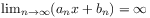thenwhile for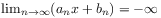follows.

[126.4.1] Suppose now that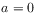. [126.4.2] Then for every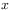and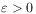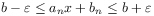(185)

[page 127, §0]    if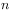is chosen sufficiently large. [127.0.1] By monotonicity of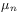it follows that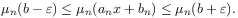(186)

[127.0.2] If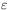is chosen so that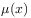is continuous at the points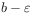and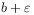, then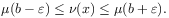(187)

[127.0.3] Becausewas arbitrary it follows that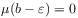and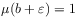. [127.0.4] Henceis degenerate, contrary to the conditions above.

[127.1.1] Finally, letbe such thatis continuous at the point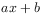, and that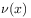is continuous at. [127.1.2] Then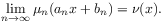(188)

[127.1.3] On the other hand because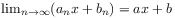one has for sufficiently largethat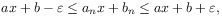(189)

whereis chosen such that the distribution function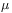is continuous at the points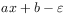and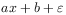. [127.1.4] Hence by monotonicity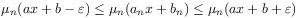(190)

and for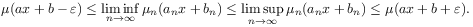(191)

[127.1.5] Becauseis a point of continuity forandis arbitrary it follows that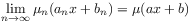(192)

and hence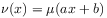proving the assertion.